# Understanding complex circuits_What to know?

#### BC547

Joined May 22, 2018
41
Reading electronic magazines ETI, Elektor, Practical Electronics,,or circuit diagrams is the best way to understand more, how each component works , like op amps, transistors, fets, putting them together and building your own circuit on Veroboard or breadboard is invaluable , .

https://www.epemag3.com/projects.html

I have a tough time understanding circuits on the outset. Capacitors are very intimidating. I have understood the basics of each discrete element, particularly capacitors, but realizing their use in a circuit is the big deal here. Any advice please?
How do you easily figure out what the circuit is doing?

#### Ian0

Joined Aug 7, 2020
5,147
I have a tough time understanding circuits on the outset. Capacitors are very intimidating. I have understood the basics of each discrete element, particularly capacitors, but realizing their use in a circuit is the big deal here. Any advice please?
How do you easily figure out what the circuit is doing?
They store charge, rather like a battery does.
If you see them with both terminals connected to the power supply, then they are just smoothing out the power supply voltage, by storing charge.
Similarly, in oscillator circuits, like the 555, the timing is determined by how long they take to charge up and discharge.

If you see them in linear circuits (audio, for example) it’s easiest to think of them as being a resistor with a resistance that varies inversely with frequency.

Does that help?

#### Wolframore

Joined Jan 21, 2019
2,541
I like the water analogy where resistors are like restrictions, capacitors are like storage tanks. There are some interesting mathematical models and properties. Do you have a grasp of inductors? Many find them just as challenging if not more so.

#### BC547

Joined May 22, 2018
41
They store charge, rather like a battery does.
If you see them with both terminals connected to the power supply, then they are just smoothing out the power supply voltage, by storing charge.
Similarly, in oscillator circuits, like the 555, the timing is determined by how long they take to charge up and discharge.

If you see them in linear circuits (audio, for example) it’s easiest to think of them as being a resistor with a resistance that varies inversely with frequency.

Does that help?
In transistor circuits, we frequently see capacitors used in conjunction with resistors. Is it all about time in such circuits?
TQ

#### Ian0

Joined Aug 7, 2020
5,147
I like the water analogy where resistors are like restrictions, capacitors are like storage tanks. There are some interesting mathematical models and properties. Do you have a grasp of inductors? Many find them just as challenging if not more so.
The water analogy works for inductors too. It’s the mass of water flowing down a pipe that an take some stopping! If you understand electronics but not plumbing, the you can reverse the analogy to understand the hydraulic ram!

#### Wolframore

Joined Jan 21, 2019
2,541
It works for inductors too but I see it as a water wheel or flywheel with a winding spring which stores energy.

#### Ian0

Joined Aug 7, 2020
5,147
In transistor circuits, we frequently see capacitors used in conjunction with resistors. Is it all about time in such circuits?
TQ
Absolutely. That is, if you want to know how the circuit behaves as time progresses.

But, You may not always want to know that. For instance, if you are designing audio, you may be less interested in how the circuit behaves over time, and more interested in its frequency response (whether it has enough treble or bass).

There are different analogies, all of which work, it’s about choosing the one that helps most.
For instance, is there a water analogy for frequency response?

Last edited:

#### Wolframore

Joined Jan 21, 2019
2,541
In transistor circuits, we frequently see capacitors used in conjunction with resistors. Is it all about time in such circuits?
TQ
Theres always a time component to RC combinations but not necessarily how you might think. Beyond the simple yes or no answer it gets complex very quickly. Capacitors being a sort of storage device can be controlled by resistors to change the fill rate... if we are still using analogies. There are many uses for components beyond the obvious ones. A common use for capacitors in transistor circuits is to pass AC variations (signal) while blocking DC. This is useful to isolate, block or get rid of AC or DC components as you might need. Again these are simplified concepts but maybe gives you an idea of few of their functions.

#### KeithWalker

Joined Jul 10, 2017
2,354
I have a tough time understanding circuits on the outset. Capacitors are very intimidating. I have understood the basics of each discrete element, particularly capacitors, but realizing their use in a circuit is the big deal here. Any advice please?
How do you easily figure out what the circuit is doing?
Understanding an undocumented complex circuit is extremely difficult and is not usually necessary, so don't worry about it. Electronic circuits usually have a descriptive title so you always have a starting point. If they are complex, they are usually broken down into functional blocks to simplify them. If a circuit uses an unusual or innovating feature, it is usually well documented.
Regards,
Keith

•BC547

#### MrChips

Joined Oct 2, 2009
26,110
Complex circuits are made up of simple circuits. Simple circuits are made up of individual components. You will be able to understand and remember what each component does if you learn about each component in the following three steps:

1 - How the component is created
2 - How the component behaves
3 - Examples of where the component is used

Capacitors

The original concept of the capacitor is the parallel plate capacitor.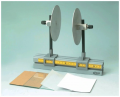In a science laboratory experiment, a parallel plate capacitor consists of two circular plates separated by some distance. The space between the plates can be any electrically insulating material, such as vacuum, air, paper, plastic, glass, etc., some of which can be seen in the foreground.

Note that in the photograph of the apparatus shown above, the circular plates are mounted on black vertical non-conducting rods. Hence the metal plates are electrically isolated from the supporting metal bench rail.

The value of the capacitance increases with the size of the plates (area) and decreases with the distance separating the plates.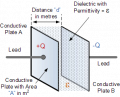Reference: https://www.electronics-tutorials.ws/capacitor/cap_1.html

The formula for capacitance of a parallel plate capacitor is: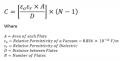As can be seen in the formula, capacitance C increases linearly with the area A (i.e. directly proportional to area A) and decreases with the distance D between the plates (i.e. inversely proportional to distance D).

Electronic symbol of a capacitor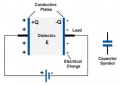You will observe that the electronic symbol of a capacitor is very appropriately drawn as two lines with a gap between the two lines. From the information presented so far, you can make the observation that a capacitor is an open circuit. No direct current can flow between the plates of the capacitor. A resistance measurement with an ohmmeter will give a reading of infinite ohms.

Properties of Capacitors

One of the early experiments with capacitors is with one known as the Leyden Jar. This consisted of a glass jar lined with lead foil on the inside and outside to form the plates of the capacitor. Early experimenters would charge the capacitor using static electricity by rubbing an ebony rod with wool or a glass rod with a silk cloth. As the story is told, in Paris in 1746, Abbé Nolle charged a Leyden jar and discharged it on a line of monks holding hands. The monks all jumped simultaneously as the electric charge traveled through their bodies.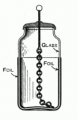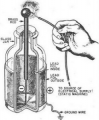Capacitors store charge

From this we observe that capacitors store electric charge.
Can capacitors serve as batteries? Yes, they can. Physical capacitance values range from 1pF to 1F which is a range of 12 orders of magnitude, 10 raised to the power 12. Capacitors with very high capacitances are not easy to make. A parallel plate air-gapped capacitor of 1F would be astronomically large and next to impossible to make. However, with latest development in dielectric materials, 1000F capacitors and even higher are now readily manufactured (albeit at low voltages, 2.7V). For obvious reasons, these are called super capacitors.

Here is a bank of 3000F 2.7V individual capacitors.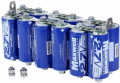An electronic photo flash requires a burst of energy at 300V in a short duration to excite the xenon flash lamp. One 1.5V AA battery cannot supply that energy continuously. However, since the photo flash is only needed once with a long resting period in between flashes a 160-330μF capacitor is charged to the required 300V from a single 1.5V battery.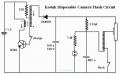Capacitors take time to charge and discharge

If you charge a capacitor with a constant current I the voltage on the capacitor rises linearly with time.
Let us look at the math.

The amount of charge (Q coulombs) placed on the capacitor is current (I amps) multiplied by time (t seconds).

Q = I x t

The attained voltage V (volts) on the capacitor is a function of the capacitance C (farads) and the charge Q.

Q = C x V

Putting the two equations together, we get
Q = I x t = C x V

or

V = I x t / C

A large capacitor takes longer to charge than a smaller capacitor.

If we were to charge the capacitor from a constant voltage, the charging current decreases (as an inverse exponential) as the difference in voltage between the source voltage Vs and the capacitor voltage Vc gets smaller. The charging current gets smaller and smaller over time. In theory, the voltage Vc on the capacitor never fully reaches the charging voltage Vs.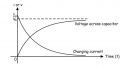The take away here is that we can apply this behaviour of a capacitor in applications that involve time, for example, time delays, pulse widths, frequency, duration, etc.

To be continued.
(I am going to put this in a blog.)

•BC547 and Wolframore

#### MrChips

Joined Oct 2, 2009
26,110
Capacitors (Part 2)

What we have learned so far is that
1) Capacitors store charge
2) Capacitors take time to charge and discharge

With these two properties, let us look at some common applications of capacitors.

The first application is in AC to DC conversion in a power supply circuit.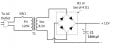Power transformer T1 steps down 120VAC to 8.5VAC. The AC signal is converted to a pulsating DC signal at twice the line frequency via the bridge rectifier B1 (which consists of four single diode rectifiers).

The waveform would look like this after rectification, shown with full-wave vs half-wave rectification.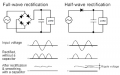The application of C1 is that of charge storage. C1 is called a reservoir capacitor. Before the advent of "super capacitors" capacitors were readily available with capacitance values in the range of 5pF to 10,000μF. We can "roughly" categorize the ranges as follows (with wide overlaps):

LOW = 1pf - 1000pF (= 1nF)
MEDIUM = 1nF - 1000nF (= 1μF)
LARGE = 1μF - 1000μF (= 1mF)
VERY LARGE = 1mF - 1000mF (= 1F)
SUPER = > 1F

C1 at 3300μF (or 3.3mF) would be considered large or very large. Its purpose is to hold the DC voltage steady at +12V. As charge is drawn from the capacitor in order to the power the load, i.e. during the discharge portion of the cycle, the voltage across the capacitor will fall. How much the voltage falls will depend on the load and the value of the capacitance. This is observed as the ripple in the power supply voltage.

The take away here is that the ripple voltage is a function of the capacitor's ability to store and deliver charge and the time in between charge and discharge portions of the AC cycle.

Let us examine a second common application of capacitors in power supply circuits.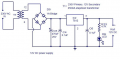In this circuit diagram of another 12VDC power supply, clearly C5, 470μF @ 25V is the reservoir capacitor.
But what is the purpose of C4 and C6, each at 0.1μF of relatively low or medium value?

We learned that the equivalent capacitance of two capacitors in parallel is the sum of each individual capacitor.
Hence,
Ceq = C5 + C4 = 470μF + 0.1μF = 470.1μF

The astute student would observe that the difference between 470μF and 470.1μF is so small that the net effect of C4 is not significant. Furthermore, the tolerance of C5 at 470μF is very likely to be ±10% or ±20% which would put the actual value of C5 to be anything from 380μF to 560μF.

*Q1 - So, that gives with C4?
*Answers to questions will be given in the final episode of this blog.

Capacitors in time-delay applications

POWER-ON RESET circuit

In many digital circuits, circuit blocks start functioning once the supply voltage exceeds a given threshold voltage. The problem with this is that some parts of the circuit may start operating before other critical parts even before the power supply voltage has stabilized. It would be preferred if circuits were started in a timely fashion in the right order. This is where the power-on reset circuit is required. This simple circuit consisting of a resistor R1 and capacitor C1 relies on the fact that the capacitor charges slowly through the current limiting resistor R1.

Before the power is applied we assume that the capacitor is in a discharged state. Thus, when power is first applied the voltage across the capacitor is close to 0V. The voltage at the -RESET pin is at logic-low which holds the applied circuit in the reset state. The capacitor slowly charges via resistor R1. In time, the voltage at the -RESET pin rises above the logic-high threshold voltage and releases the circuit from its reset state. The time taken for this power-on delay is approximately equal to the number of seconds calculated by R1 (ohms) x C1 (farads). This RC value is known as the RC time-constant and you will see this appearing frequently in circuit design applications.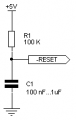Delays, one-shot circuits, square-wave generators

With the knowledge that an RC circuit produces a voltage signal that rises or falls slowly, we can apply this in order to design circuits that produces delays, timed pulses, waveform generators.

Since a capacitor takes time to charge and discharge, we can use this property to slow down the rising or falling edge of a signal.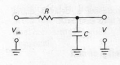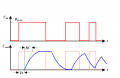We can use this to generate one-shot pulses or a continuous square-wave.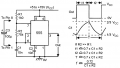(Work in progress)
*Answers to questions will be given in the final episode of this blog.

Still to come:
Capacitors as integrators and differentiators.
Capacitors as DC blocking capacitors in inter-stage audio components
Capacitors in AC circuits, low-pass and high pass filters, tone control circuits
Capacitors in RF tuned circuits, variable capacitors

Last edited:

#### dl324

Joined Mar 30, 2015
14,466
In transistor circuits, we frequently see capacitors used in conjunction with resistors. Is it all about time in such circuits?
It depends on the circuit. It could be to insert a zero (more gain) in the frequency response, speed up response (speed-up capacitor), or decouple noise. We need to see a schematic to determine why it's being used.

#### nsaspook

Joined Aug 27, 2009
9,731
In transistor circuits, we frequently see capacitors used in conjunction with resistors. Is it all about time in such circuits?
TQ
Many times in circuits electronic (non-tuning) capacitors are used as a special type of wire in addition to RC time related circuit functions. We use a value of capacitance where the reactive effect to the passing signal is minimal in effect to the signal. When this happens the capacitor in effect becomes a special wire that passes (couples) changing signals but stops steady state signals or voltages.
https://eepower.com/capacitor-guide/applications/coupling-and-decoupling/#

It becomes a strange "conductor" where the two terminals are connected by electrical forces which reach across a narrow space. It is not a true conductor, but neither is it a simple insulator.

#### MrChips

Joined Oct 2, 2009
26,110
Capacitors (Part 3)

Integrators and differentiators

We have discovered that capacitors store electric charge, in other words, a capacitor accumulates any charge presented to it.
A capacitor integrates (sums) current (charge). The voltage rises as more current (charge) is received. Mathematically, a capacitor performs the mathematical integration of current.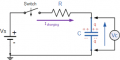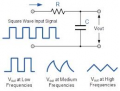If we interchange R and C we get a different effect. Remember, no DC current can flow across the plates of a capacitor. However, the resistor responds to the rate of change of the input voltage.Whereas the previous configuration represents a mathematical integration of current, this configuration represents a mathematical differentiation of voltage. If you are uncertain of the mathematical significance of integration and differentiation you may want to review any basic level course in Calculus.

We can use these properties of a capacitor to perform analog integration and differentiation.

Op-amp integrator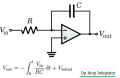Op-amp differentiator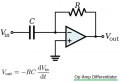Low pass and high pass filters

It may come as a surprise to you that an R-C integrator is a low pass filter, while a C-R differentiator is a high pass filter.
Let us study these one at a time.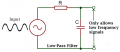We keep returning to the common theme of the fact that a capacitor stores charge and that it takes time for the capacitor to charge and discharge. What this means is that the voltage across the capacitor changes slowing and therefore has a damping or smoothing effect. It tries to keep the voltage steady. High frequency signals get attenuated. Low frequency signals are less affected. There must be a transition band between low frequencies with little attenuation and high frequencies that get attenuated. This comes back to the concept of RC time-constant which is the product of R x C.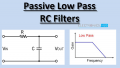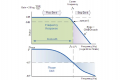Amazingly, the critical frequency is equal to 1/RC

transition frequency ω (radians/second) = 1 / RC

ω = 2πf = 1 / RC

That critical frequency is called the corner frequency or cutoff frequency fc and can be determined by the formula

fc = 1 / ( 2πRC)

where
fc = cutoff frequency in Hz
R = series resistance in ohm
C = parallel capacitance in Farad

For example,
R = 1kΩ
C = 1μF
fc = 159Hz

There are may online low pass filter calculators which will allow you to select R and C values in order to determine the cutoff frequency. Conversely you can select any two of the three parameters, R, C, and F, and determine the unknown third parameter.

Here is one example: